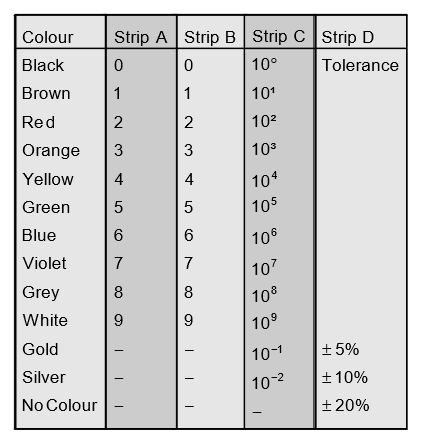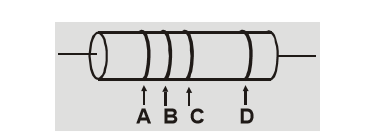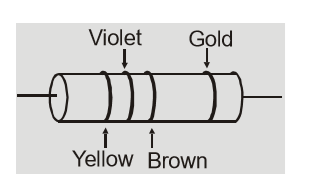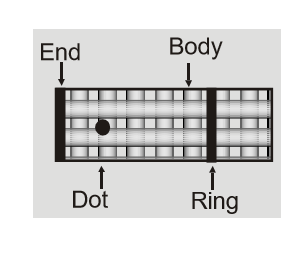Most Affordable JEE | NEET | 8,9,10 Preparation by Kota's Top IITian Doctor Faculties

# How to read a resistance color code?Resistors are the most common and important component of any electronic circuit. In daily life we see a lot of circuits having resistances but how do we recognize them? To know the value of a resistance they are color coded with rings which have specific meanings according to the color and the place they are at. Let's figure out now what Resistance Color Code is and what it all means!! Contents:

## Resistance Color Code

Resistors are produced for laboratory and domestic uses. Generally they are available in two types:
1. Wire bound Resistors
2. Carbon Resistors
Wire bound resistors are made up by winding the alloy such as Nichrome, Manganin, Constantan etc as their resistivities are relatively insensitive to the temperature. they can be in the range of a fraction of an ohm to few hundred ohms. The second one, Carbon Resistors are used to make higher range of resistors. These are made up of Carbon. These are in general compact, inexpensive hence used extensively in electronic circuits. These are very small so their values are given by color coding them. Next we are going to see what is this color coding.

## Color coding of Carbon Resistors

Check out the following table where each color is followed by 4 Strips. Here we will see what those 4 colored rings mean wound around a resistor. [caption id="attachment_4578" align="aligncenter" width="483"]Carbon Resistance Color Code Table[/caption]

### Mnemonics

A useful mnemonic matches the first letter of the color code, in numeric order. Here are two that includes tolerance codes gold, silver, and none:
• Bad beer rots our young guts but vodka goes well – get some now.
• B B ROY of Great Britain had a Very Good Wife who wore Gold and Silver Necklace.
[source] A Carbon resistor looks like the one shown above. It has 4 rings around it. [caption id="attachment_4579" align="aligncenter" width="308"]Carbon Resistance Color Code[/caption] The first two rings give first two significant figures of resistance. The third ring indicates the decimal multiplier i.e. the number of zeroes that will follow the two significant figures. The fourth ring shows tolerance or percentage accuracy.
Q.What will be the color code for $42{\rm{k}}\Omega \pm 10\%$ carbon resistance.
Ans.According to color code color for digit 4 is yellow, for digit 2 it is red, for 3 colors is orange and 10$\%$ tolerance is represented by silver color. So color code should be yellow, red, orange and silver.
Q.What is resistance of following resistor?Ans.Brown color gives multiplier ${10^\prime },$ Gold gives a tolerance of $\pm 5\%$ So resistance of resistor is $47 \times {10^1}\Omega \pm 5\% \quad$ or $\quad 470 \pm 5\% \Omega$

## Body End Dot Color Code

Earlier, resistors were manufactured with this color code. [caption id="attachment_4581" align="aligncenter" width="217"]Body End Dot Color Code[/caption]
1. The color of body gives first significant figure.
2. The color of end gives second significant figure.
3. The color of dot gives the decimal multiplier.
4. The color of ring gives the tolerance.